Conditional Probability,The Monty Hall Problem

Sometimes we already know the ocurrence of an event A, then the probability of a relevent event B given A is different from P(B) without any information on A. Since the sample space is reducedd from the total space to A and the probability that B will occur given that A has occured is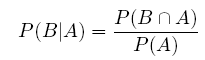Example: Suppose we throw two fair dices. Consider the following three events:

A: Dice 1 lands on 3

B: Dice 2 lands on 1

C: The sum is 8

First notice that C= {(2,6),(3,5),(4,4),(5,3),(6,2)}, and the total number of possible outcomes rolling two dices is 6*6=36, therefore P(C)=5/36. Obviously, P(A)=1/6 and P(B)=1/6. Sometimes this is called the priority probability of each event.

Next, we have 'Both A and C occur'={(3,5)} , 'Both B and C occur'=empty and 'Both A and B occur'={(3,1)}. The probability of events occuring together is called joint probability. We have P(A intersects C)=1/36 , P(B intersects C)=0 and P(A intersects B)=1/36.

Then you can compute all the conditional probabilities using the priority probability and joint probability given above.

Notice that we haveWhen the above equation holds for two events A and B, we say they are (statistically) independent. Intuitively, this means that the two events don't interfere with each other. Equivalently, independence means that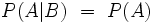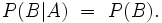As the last example may have suggested, the mapping from event B to conditional probability of B given A (A a fixed event) is a probability. You may look up the axioms of probability and check the conditions one by one.

You may wish to try the next problem by yourself:

Problem: Anne and Billy are playing a simple dice game. Each rolls one dice and the one with higher number wins. If the numbers are the same, they roll again. If Anne just won, what is the probability that she rolled a '5'?

Let A denote the event that 'Anne wins'. If we write out the outcomes with Anne's roll first and Billy's roll second, the event A is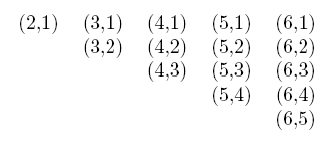There are 5+4+3+2+1=15 outcomes in event A and the event 'Anne rolled a 5 and won' has 4 outcomes as shown clearly in the above table. Therefore the joint probability is 4/36 and P(A)=15/36, then we have the conditional probability is 4/15.

Conditional probability can be very puzzling sometimes, actually it is the sourse of many 'paradoxes' in probability. One of these attracted worldwide attention in 1990 when Marilyn vos Savant discussed it in her weekly column in the Sunday Parade magazine.

The Monty Hall Problem:

The statement of this famous problem in Parade Magazine is as follows:

Suppose you're on a game show, and you're given the choice of three doors: Behind one door is a car; behind the others, donkey. You pick a door, say No.1, and the host, who knows what's behind the doors, opens another door, say No.3, which has a donkey. He then says to you, "Do you want to pick door No.2?" Is it to your advantage to switch your choice? (Whitaker 1990)

Many people argue that "the two unopened doors are the same so they each will contain the car with probability 1/2, and hence there is no point in switching." As we will now show, this naive reasoning is incorrect. To compute the answer, we will suppose that the host always chooses to show you a donkey (When the problem and the solution appeared in Parade, approximately 10,000 readers, including nearly 1,000 with Ph.D.s, wrote to the magazine claiming the published solution was wrong. Some of the controversy was because the Parade version of the problem is technically ambiguous since it leaves certain aspects of the host's behavior unstated, for example whether the host must open a door and must make the offer to switch).

Assuming that you have picked door No.1, there are 3 cases: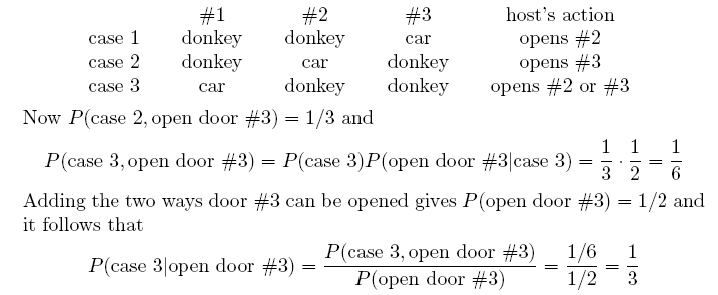Notice that although it took a number of steps to compute this answer, it is "obvious". When we picked one of the three doors initially we had probability 1/3 of picking the car, and since the host can always open a door with a donkey the new information does not change our chance of winning.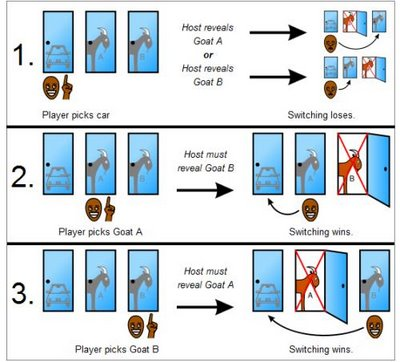The above argument may easily persuade you in a moment by when you think about it again by yourself, it is still somewhat confusing. So why is it so confusing?

Source of Confusion:

When first presented with the Monty Hall problem an overwhelming majority of people assume that each door has an equal probability and conclude that switching does not matter (Mueser and Granberg, 1999). Out of 228 subjects in one study, only 13% chose to switch (Granberg and Brown, 1995:713).

In her book The Power of Logical Thinking, vos Savant (1996:15) quotes cognitive psychologist Massimo Piattelli-Palmarini as saying "... no other statistical puzzle comes so close to fooling all the people all the time" and "that even Nobel physicists systematically give the wrong answer, and that they insist on it, and they are ready to berate in print those who propose the right answer."

Most statements of the problem, notably the one in Parade Magazine, do not match the rules of the actual game show (Krauss and Wang, 2003:9), and do not fully specify the host's behavior or that the car's location is randomly selected (Granberg and Brown, 1995:712). Krauss and Wang (2003:10) conjecture that people make the standard assumptions even if they are not explicitly stated. Although these issues are mathematically significant, even when controlling for these factors nearly all people still think each of the two unopened doors has an equal probability and conclude switching does not matter (Mueser and Granberg, 1999). This "equal probability" assumption is a deeply rooted intuition (Falk 1992:202). People strongly tend to think probability is evenly distributed across as many unknowns as are present, whether it is or not (Fox and Levav, 2004:637).

A competing deeply rooted intuition at work in the Monty Hall problem is the belief that exposing information that is already known does not affect probabilities (Falk 1992:207). This intuition is the basis of solutions to the problem that assert the host's action of opening a door does not change the player's initial 1/3 chance of selecting the car. For the fully explicit problem this intuition leads to the correct numerical answer, 2/3 chance of winning the car by switching, but leads to the same solution for other variants where this answer is not correct (Falk 1992:207).

Another source of confusion is that the usual wording of the problem statement asks about the conditional probability of winning given which door is opened by the host, as opposed to the overall or unconditional probability. These are mathematically different questions and can have different answers depending on how the host chooses which door to open if the player's initial choice is the car (Morgan et al., 1991; Gillman 1992). For example, if the host opens Door 3 whenever possible then the probability of winning by switching for players initially choosing Door 1 is 2/3 overall, but only 1/2 if the host opens Door 3. In its usual form the problem statement does not specify this detail of the host's behavior, making the answer that switching wins the car with probability 2/3 mathematically unjustified. Many commonly presented solutions address the unconditional probability, ignoring which door the host opens; Morgan et al. call these "false solutions" (1991).

There are various version of the Monte Hall problem developed after the original Parade version.

Example:Cognitive dissonance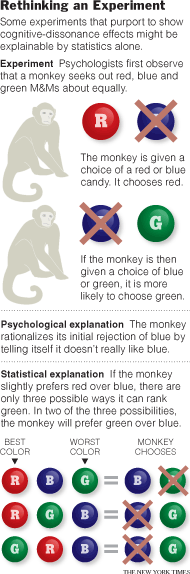An economist, M. Keith Chen, has recently uncovered a version of the Monty Hall problem in the theory of cognitive dissonance. For a half-century, experimenters have been using the so-called free choice paradigm to test our tendency to rationalize decisions. In an experiment typical of the genre, Yale psychologists measured monkeys preferences by observing how quickly each monkey sought out different colors of M&Ms.

In the first step, the researchers gave the monkey a choice between say red and blue. If the monkey chose red, then it was given a choice between blue and green. Nearly two-thirds of the time it rejected blue in favor of green, which seemed to jibe with the theory of choice rationalization: once we reject something, we tell ourselves we never liked it anyway.

Putting aside this interpretation it is natural to ask: What would happen if monkeys were acting at random? The six orderings RGB, RBG, GRB, GBR, BGR, and BRG would have equal probability. In the ?rst three cases red is preferred to blue, but in 2/3s of those cases green is preferred to blue. Just as in the Monty Hall problem, we think that the probability of preferring blue to green is 1/2 due to symmetry, but the probability is 1/3. This time however conditioning on red being preferred to green reduced the original probability of 1/2 to 1/3, whereas in the Monty Hall problem the probability was initially 1/3 and did not change.